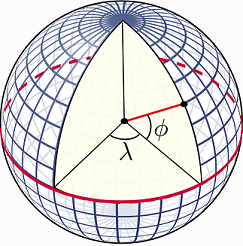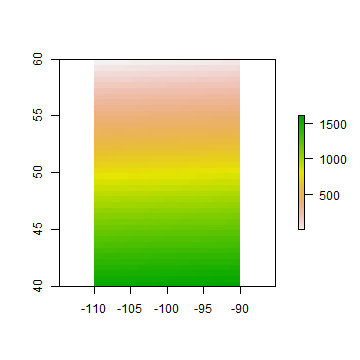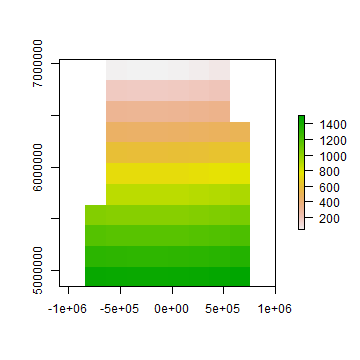# Coordinate Reference Systems¶

## Introduction¶

A very important aspect of spatial data is the coordinate reference system (CRS) that is used. For example, a location of (140, 12) is not meaningful if you do know where the origin is and if the x-coordinate is 140 meters, kilometers, or perhaps degrees away from it (in the x direction).

## Coordinate Reference Systems¶

### Angular coordinates¶

The earth has an irregular spheroid-like shape. The natural coordinate reference system for geographic data is longitude/latitude. This is an angular system. The latitude (phi) of a point is the angle between the equatorial plane and the line that passes through a point and the center of the Earth. Longitude (lambda) is the angle from a reference meridian (lines of constant longitude) to a meridian that passes through the point.Obviously we cannot actually measure these angles. But we can estimate them. To do so, you need a model of the shape of the earth. Such a model is called a ‘datum’. The simplest datums are a spheroid (a sphere that is ‘flattened’ at the poles and bulges at the equator). More complex datums allow for more variation in the earth’s shape. The most commonly used datum is called WGS84 (World Geodesic System 1984). This is very similar to NAD83 (The North American Datum of 1983). Other, local datums exist to more precisely record locations for a single country or region.

So the basic way to record a location is a coordinate pair in degrees and a reference datum. (Sometimes people say that their coordinates are “in WGS84”. That is meaningless; but they typically mean to say that they are longitude/latitude relative to the WGS84 datum).

### Projections¶

A major question in spatial analysis and cartography is how to transform this three dimensional angular system to a two dimensional planar (sometimes called “Cartesian”) system. A planar system is easier to use for certain calculations and required to make maps (unless you have a 3-d printer). The different types of planar coordinate reference systems are referred to as ‘projections’. Examples are ‘Mercator’, ‘UTM’, ‘Robinson’, ‘Lambert’, ‘Sinusoidal’ ‘Robinson’ and ‘Albers’.

There is not one best projection. Some projections can be used for a map of the whole world; other projections are appropriate for small areas only. One of the most important characteristics of a map projection is whether it is “equal area” (the scale of the map is constant) or “conformal” (the shapes of the geographic features are as they are seen on a globe). No two dimensional map projection can be both conformal and equal-area (but they can be approximately both for smaller areas, e.g. UTM, or Lambert Equal Area for a larger area), and some are neither.

### Notation¶

A planar CRS is defined by a projection, datum, and a set of parameters. The parameters determine things like where the center of the map is. The number of parameters depends on the projection. It is therefore not trivial to document a projection used, and several systems exist. In R we use the [PROJ.4[(ftp://ftp.remotesensing.org/proj/OF90-284.pdf ) notation. PROJ.4 is the name of an open source software library that is commonly used for CRS transformation.

Here is a list of commonly used projections and their parameters in PROJ4 notation. You can find many more of these on spatialreference.org

Most commonly used CRSs have been assigned a “EPSG code” (EPSG stands for European Petroleum Survey Group). This is a unique ID that can be a simple way to identify a CRS. For example `EPSG:27561` is equivalent to `+proj=lcc +lat_1=49.5 +lat_0=49.5 +lon_0=0 +k_0=0.999877341 +x_0=6 +y_0=2 +a=6378249.2 +b=6356515` `+towgs84=-168,-60,320,0,0,0,0 +pm=paris +units=m +no_defs`. However `EPSG:27561` is opaque and should not be used outside of databases. In R use the PROJ.4 notation, as that can be readily interpreted without relying on software.

Below is an illustration of how to find a particular projection you may need (in this example, a list of projections for France).

```library(rgdal)
epsg <- make_EPSG()
i <- grep("France", epsg\$note, ignore.case=TRUE)
# first three
epsg[i[1:3], ]
##       code                                note
## 1399  2192           ED50 / France EuroLambert
## 2466 27561   NTF (Paris) / Lambert Nord France
## 2467 27562 NTF (Paris) / Lambert Centre France
##                                                                                                                                            prj4
## 1399 +proj=lcc +lat_1=46.8 +lat_0=46.8 +lon_0=2.33722916666667 +k_0=0.99987742 +x_0=600000 +y_0=2200000 +ellps=intl +units=m +no_defs +type=crs
## 2466 +proj=lcc +lat_1=49.5 +lat_0=49.5 +lon_0=0 +k_0=0.999877341 +x_0=600000 +y_0=200000 +ellps=clrk80ign +pm=paris +units=m +no_defs +type=crs
## 2467  +proj=lcc +lat_1=46.8 +lat_0=46.8 +lon_0=0 +k_0=0.99987742 +x_0=600000 +y_0=200000 +ellps=clrk80ign +pm=paris +units=m +no_defs +type=crs
##                         prj_method
## 1399 Lambert Conic Conformal (1SP)
## 2466 Lambert Conic Conformal (1SP)
## 2467 Lambert Conic Conformal (1SP)
```

Now let’s look at an example with a spatial data set in R.

```library(raster)
library(rgdal)
f <- system.file("external/lux.shp", package="raster")
p <- shapefile(f)
p
## class       : SpatialPolygonsDataFrame
## features    : 12
## extent      : 5.74414, 6.528252, 49.44781, 50.18162  (xmin, xmax, ymin, ymax)
## crs         : +proj=longlat +datum=WGS84 +no_defs
## variables   : 5
## names       : ID_1,     NAME_1, ID_2,   NAME_2, AREA
## min values  :    1,   Diekirch,    1, Capellen,   76
## max values  :    3, Luxembourg,   12,    Wiltz,  312
```

We can inspect the coordinate reference system like this.

```crs(p)
## Coordinate Reference System:
## Deprecated Proj.4 representation: +proj=longlat +datum=WGS84 +no_defs
## WKT2 2019 representation:
## GEOGCRS["WGS 84",
##     DATUM["World Geodetic System 1984",
##         ELLIPSOID["WGS 84",6378137,298.257223563,
##             LENGTHUNIT["metre",1]]],
##     PRIMEM["Greenwich",0,
##         ANGLEUNIT["degree",0.0174532925199433]],
##     CS[ellipsoidal,2],
##         AXIS["latitude",north,
##             ORDER,
##             ANGLEUNIT["degree",0.0174532925199433]],
##         AXIS["longitude",east,
##             ORDER,
##             ANGLEUNIT["degree",0.0174532925199433]],
##     ID["EPSG",4326]]
```

## Assigning a CRS¶

Sometimes we have data without a CRS. This can be because the file used was incomplete, or perhaps because we created the data ourselves with R code. In that case we can assign the CRS if we know what it should be. Here I first remove the CRS of `pp` and then I set it again.

```pp <- p
crs(pp) <- NA
crs(pp)
## Coordinate Reference System:
## Deprecated Proj.4 representation: NA
crs(pp) <- CRS("+proj=longlat +datum=WGS84")
crs(pp)
## Coordinate Reference System:
## Deprecated Proj.4 representation: +proj=longlat +datum=WGS84 +no_defs
## WKT2 2019 representation:
## GEOGCRS["unknown",
##     DATUM["World Geodetic System 1984",
##         ELLIPSOID["WGS 84",6378137,298.257223563,
##             LENGTHUNIT["metre",1]],
##         ID["EPSG",6326]],
##     PRIMEM["Greenwich",0,
##         ANGLEUNIT["degree",0.0174532925199433],
##         ID["EPSG",8901]],
##     CS[ellipsoidal,2],
##         AXIS["longitude",east,
##             ORDER,
##             ANGLEUNIT["degree",0.0174532925199433,
##                 ID["EPSG",9122]]],
##         AXIS["latitude",north,
##             ORDER,
##             ANGLEUNIT["degree",0.0174532925199433,
##                 ID["EPSG",9122]]]]
```

Note that you should not use this approach to change the CRS of a data set from what it is to what you want it to be. Assigning a CRS is like labeling something. You need to provide the label that corresponds to the item. Not to what you would like it to be. For example if you label a bicycle, you can write “bicycle”. Perhaps you would prefer a car, and you can label your bicycle as “car” but that would not do you any good. It is still a bicycle. You can try to transform your bicycle into a car. That would not be easy. Transforming spatial data is easier.

## Transforming vector data¶

We can transform these data to a new data set with another CRS using the `spTransform` function from the `rgdal` package.

Here we use the Robinson projection. First we need to find the correct notation.

```newcrs <- CRS("+proj=robin +datum=WGS84")
```

Now use it

```rob <- spTransform(p, newcrs)
rob
## class       : SpatialPolygonsDataFrame
## features    : 12
## extent      : 471320.7, 536010.5, 5269709, 5345677  (xmin, xmax, ymin, ymax)
## crs         : +proj=robin +lon_0=0 +x_0=0 +y_0=0 +datum=WGS84 +units=m +no_defs
## variables   : 5
## names       : ID_1,     NAME_1, ID_2,   NAME_2, AREA
## min values  :    1,   Diekirch,    1, Capellen,   76
## max values  :    3, Luxembourg,   12,    Wiltz,  312
```

After the transformation, the units of the geometry are no longer in degrees, but in meters away from (longitude=0, latitude=0). The spatial extent of the data is also in these units.

We can backtransform to longitude/latitude:

```p2 <- spTransform(rob, CRS("+proj=longlat +datum=WGS84"))
```

## Transforming raster data¶

Vector data can be transformed from lon/lat coordinates to planar and back without loss of precision. This is not the case with raster data. A raster consists of rectangular cells of the same size (in terms of the units of the CRS; their actual size may vary). It is not possible to transform cell by cell. Rather estimates for the values of new cells must be made based on the values in the old cells. If the values are class data, the ‘nearest neighbor’ is commonly used. Otherwise some sort of interpolation (e.g. ‘bilinear’).

Because projection of rasters affects the cell values, in most cases you will want to avoid projecting raster data and rather project vector data. But when you do project raster data, you want to assure that you project to exactly the raster definition you need (so that it lines up with other raster data you are using).

```r <- raster(xmn=-110, xmx=-90, ymn=40, ymx=60, ncols=40, nrows=40)
r <- setValues(r, 1:ncell(r))
r
## class      : RasterLayer
## dimensions : 40, 40, 1600  (nrow, ncol, ncell)
## resolution : 0.5, 0.5  (x, y)
## extent     : -110, -90, 40, 60  (xmin, xmax, ymin, ymax)
## crs        : +proj=longlat +datum=WGS84 +no_defs
## source     : memory
## names      : layer
## values     : 1, 1600  (min, max)
plot(r)
```Here is a new PROJ4 projection description.

```newproj <- "+proj=lcc +lat_1=48 +lat_2=33 +lon_0=-100 +ellps=WGS84"
```

Simplest approach

```pr1 <- projectRaster(r, crs=newproj)
crs(pr1)
## Coordinate Reference System:
## Deprecated Proj.4 representation:
##  +proj=lcc +lat_0=0 +lon_0=-100 +lat_1=48 +lat_2=33 +x_0=0 +y_0=0
## +ellps=WGS84 +units=m +no_defs
## WKT2 2019 representation:
## PROJCRS["unknown",
##     BASEGEOGCRS["unknown",
##         DATUM["Unknown based on WGS84 ellipsoid",
##             ELLIPSOID["WGS 84",6378137,298.257223563,
##                 LENGTHUNIT["metre",1],
##                 ID["EPSG",7030]]],
##         PRIMEM["Greenwich",0,
##             ANGLEUNIT["degree",0.0174532925199433],
##             ID["EPSG",8901]]],
##     CONVERSION["unknown",
##         METHOD["Lambert Conic Conformal (2SP)",
##             ID["EPSG",9802]],
##         PARAMETER["Latitude of false origin",0,
##             ANGLEUNIT["degree",0.0174532925199433],
##             ID["EPSG",8821]],
##         PARAMETER["Longitude of false origin",-100,
##             ANGLEUNIT["degree",0.0174532925199433],
##             ID["EPSG",8822]],
##         PARAMETER["Latitude of 1st standard parallel",48,
##             ANGLEUNIT["degree",0.0174532925199433],
##             ID["EPSG",8823]],
##         PARAMETER["Latitude of 2nd standard parallel",33,
##             ANGLEUNIT["degree",0.0174532925199433],
##             ID["EPSG",8824]],
##         PARAMETER["Easting at false origin",0,
##             LENGTHUNIT["metre",1],
##             ID["EPSG",8826]],
##         PARAMETER["Northing at false origin",0,
##             LENGTHUNIT["metre",1],
##             ID["EPSG",8827]]],
##     CS[Cartesian,2],
##         AXIS["(E)",east,
##             ORDER,
##             LENGTHUNIT["metre",1,
##                 ID["EPSG",9001]]],
##         AXIS["(N)",north,
##             ORDER,
##             LENGTHUNIT["metre",1,
##                 ID["EPSG",9001]]]]
```

Alternatively, you can also set the resolution.

```pr2 <- projectRaster(r, crs=newproj, res=20000)
pr2
## class      : RasterLayer
## dimensions : 124, 94, 11656  (nrow, ncol, ncell)
## resolution : 20000, 20000  (x, y)
## extent     : -944881.5, 935118.5, 4664378, 7144378  (xmin, xmax, ymin, ymax)
## crs        : +proj=lcc +lat_0=0 +lon_0=-100 +lat_1=48 +lat_2=33 +x_0=0 +y_0=0 +ellps=WGS84 +units=m +no_defs
## source     : memory
## names      : layer
## values     : -16.22972, 1616.249  (min, max)
```

But to have more control, provide an existing Raster object. That is generally the best way to project raster. By providing an existing Raster object, such that your newly projected data perfectly aligns with it. In this example we do not have an existing Raster object, so we create one using `projectExtent`.

```pr3 <- projectExtent(r, newproj)
# Set the cell size
res(pr3) <- 200000
```

Now project, and note the change in the coordinates.

```pr3 <- projectRaster(r, pr3)
pr3
## class      : RasterLayer
## dimensions : 11, 8, 88  (nrow, ncol, ncell)
## resolution : 2e+05, 2e+05  (x, y)
## extent     : -844881.5, 755118.5, 4844378, 7044378  (xmin, xmax, ymin, ymax)
## crs        : +proj=lcc +lat_0=0 +lon_0=-100 +lat_1=48 +lat_2=33 +x_0=0 +y_0=0 +ellps=WGS84 +units=m +no_defs
## source     : memory
## names      : layer
## values     : 41.84528, 1503.516  (min, max)
plot(pr3)
```For raster based analysis it is often important to use equal area projections, particularly when large areas are analyzed. This will assure that the grid cells are all of same size, and therefore comparable to each other.